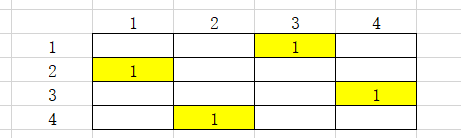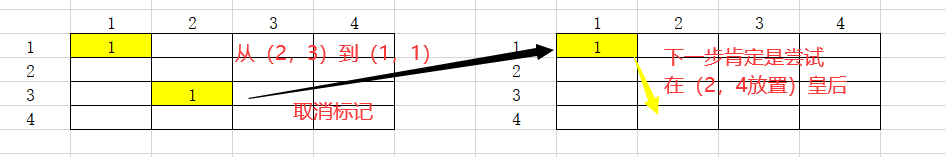# 分析• one：要判断我们放的皇后是不是共线，我们可以对以前放置的皇后的所在的行和对角线(这里就用到了坐标与对角线的关系)进行标记。
• two：我们在不断的尝试放置皇后时，要回到上一层，肯定要取消掉当前点的标记。取消当前点的标记并返回上一层的这个过程就叫回溯，回溯可以避免上一次的标记对下面搜索的影响。
eg：在 4 * 4 的棋盘中，当我们标记完（2，3）时，发现第三列根本无法放置皇后，我们就要回到上一层的（1，1）。这时我们就要去掉（2，3）这个点的标记，到（1，1）去尝试向第二列的其他点放置皇后。# 代码

``````#include <bits/stdc++.h>
using namespace std;

int hang = {0};	// 标记行
int zhu  = {0};	// 标记主对角线
int ci  = {0};	// 标记次对角线
int res;		// 存每组的坐标
int num = 0;		// 输出坐标组数
int ans = 0;		// 方案总数
int n;

void pr(){		//输出前三组的坐标
num++;
for(int i = 1; i <= n; i++) cout << res[i] << " ";
cout << endl;
}

void DFS(int x){
if(x > n){				// 找到如果走到第 n+1 列，说明前 n 列已经都放下了皇后
ans++;				// 方案数 +1
if(num < 3) pr();		// 看是不是前三组答案
return;
}

for(int y = 1; y <= n; y++){
if(hang[y]) continue;				// 同一行
if(zhu[x - y + n]) continue;			// 同一条主对角线
if(ci[x + y]) continue;				// 同一条次对角线
hang[y] = zhu[x - y + n] = ci[x + y] = 1;	// 标记这个点在的行和对角线
res[x] = y;					// 纪录这个点
DFS(x+1);					// 搜索
hang[y] = zhu[x - y + n] = ci[x + y] = 0;	// 回溯
}
}

int main() {

ios::sync_with_stdio(false);

cin >> n;

DFS(1);		// 从第一列开始搜索

cout << ans << endl;

return 0;
}``````